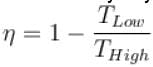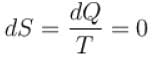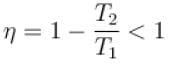Second Law Of Termodynamics – MSQ

# Second Law Of Termodynamics – MSQ

Test Description

## 10 Questions MCQ Test Topic wise Tests for IIT JAM Physics | Second Law Of Termodynamics – MSQ

Second Law Of Termodynamics – MSQ for Physics 2022 is part of Topic wise Tests for IIT JAM Physics preparation. The Second Law Of Termodynamics – MSQ questions and answers have been prepared according to the Physics exam syllabus.The Second Law Of Termodynamics – MSQ MCQs are made for Physics 2022 Exam. Find important definitions, questions, notes, meanings, examples, exercises, MCQs and online tests for Second Law Of Termodynamics – MSQ below.
Solutions of Second Law Of Termodynamics – MSQ questions in English are available as part of our Topic wise Tests for IIT JAM Physics for Physics & Second Law Of Termodynamics – MSQ solutions in Hindi for Topic wise Tests for IIT JAM Physics course. Download more important topics, notes, lectures and mock test series for Physics Exam by signing up for free. Attempt Second Law Of Termodynamics – MSQ | 10 questions in 45 minutes | Mock test for Physics preparation | Free important questions MCQ to study Topic wise Tests for IIT JAM Physics for Physics Exam | Download free PDF with solutions
 1 Crore+ students have signed up on EduRev. Have you?
*Multiple options can be correct
Second Law Of Termodynamics – MSQ - Question 1

### Which of the following is/are correct statement? Select one or more:

Detailed Solution for Second Law Of Termodynamics – MSQ - Question 1

All reversible engines have same efficiency only if they operate between same temperature and is given byThe correct answer is: All the reversible engines operating between same temperatures have same efficiency

*Multiple options can be correct
Second Law Of Termodynamics – MSQ - Question 2

### Entropy does not remains constant in which of the following processes? Select one or more:

Detailed Solution for Second Law Of Termodynamics – MSQ - Question 2

In adiabatic process, dQ = 0
⇒⇒ S remains constant.
The correct answers are: Isothermal process, Cyclic process, Isobaric process

*Multiple options can be correct
Second Law Of Termodynamics – MSQ - Question 3

### The main cause of irreversibility is/are. Select one or more:

Detailed Solution for Second Law Of Termodynamics – MSQ - Question 3

All the 3 options (a, b & c) results in the loss of heat and thus, result in irreversibility.
The correct answers are: mechanical and fluid friction, unrestricted expansion, heat transfer with a finite temperature difference

*Multiple options can be correct
Second Law Of Termodynamics – MSQ - Question 4

Which of the following is a property of entropy?
Select one or more:

Detailed Solution for Second Law Of Termodynamics – MSQ - Question 4

From second law of thermodynamics, (a) and (b) are true.
In adiabatic process, dQ = 0
⇒ dS = 0, hence (c) is also true.
The correct answers are: Entropy increases during an irreversible operation   , Net change in entropy in a reversible cycle is zero, Change in entropy during an adiabatic operation is zero

*Multiple options can be correct
Second Law Of Termodynamics – MSQ - Question 5

A Carnot cycle operates on a working substance between two reservoirs at temperature T1 and T2, where T1 >T2. During each cycle, an amount of heat Q1 is extracted from the reservoir at T1 and an amount Q2 is delivered to the reservoir at T2. Which of the following statements are correct?
Select one or more:

Detailed Solution for Second Law Of Termodynamics – MSQ - Question 5

Here, the only false statement is efficiency is equal to 1
∴The correct answers are: work done in one cycle is Q1 – Q2, entropy of the hotter reservoir decreases, entropy for the universe (consisting of the working substance and the two reservoirs) increases

*Multiple options can be correct
Second Law Of Termodynamics – MSQ - Question 6

The condition for the reversibility of a cycle is.
Select one or more:

Detailed Solution for Second Law Of Termodynamics – MSQ - Question 6

All the four options given above are necessary for reversibility of a cycle

The correct answers are: The pressure and temperature of working substance must not differ, appreciably from those of the surroundings at any stage in the process, All the processes taking place in the cycle of operation, must be extremely slow, The working parts of the engine must be friction free, There should be no loss of energy during the cycle of operation

*Multiple options can be correct
Second Law Of Termodynamics – MSQ - Question 7

Which of the following statements are correct?
Select one or more:

Detailed Solution for Second Law Of Termodynamics – MSQ - Question 7

(a, b, c) are all different definitions associated with the entropy and are thus all correct
The correct answers are: The increase in entropy is obtained from a given quantity of heat at a low temperature, The change in entropy may be regarded as a measure of the rate of the availability of heat for transformation into work., The entropy represents the maximum amount of work obtainable per degree drop in temperature

*Multiple options can be correct
Second Law Of Termodynamics – MSQ - Question 8

For any process, the second law of thermodynamics requires that the change of entropy of the universe be.
Select one or more:

Detailed Solution for Second Law Of Termodynamics – MSQ - Question 8

In reversible processes, change in entropy is zero but in irreversible process, change in entropy is positive. So, for any process, the change of entropy of the universe will be positive or zero.
The correct answers are: positive, zero

*Multiple options can be correct
Second Law Of Termodynamics – MSQ - Question 9

Out of three Carnot engines, operating between reservoir temperature of (a) 400K and 500K (b) 600K and 800K (c) 800K and 1000K, which has/have the lowest thermal efficiency?
Select one or more:

Detailed Solution for Second Law Of Termodynamics – MSQ - Question 9

n=1-(TL/Tn)

For(a), n=1-(400/500) =1/5=0.2

For(b), n=1-(600/800) =1/4=0.25

For(c), n=1-(800/1000) =1/5=0.2

The option B and C is the correct answer.

*Multiple options can be correct
Second Law Of Termodynamics – MSQ - Question 10

According to Carnot’s theorem.
Select one or more:

Detailed Solution for Second Law Of Termodynamics – MSQ - Question 10

Efficiency of Carnot engine doesn’t depend on working substance and its efficiency is always less than unity.
The correct answers are: Efficiency of a Carnot engine solely depends on hot and cold temperature reservoirs, Carnot engine has the maximum possible efficiency

## Topic wise Tests for IIT JAM Physics

217 tests
 Use Code STAYHOME200 and get INR 200 additional OFF Use Coupon Code
Information about Second Law Of Termodynamics – MSQ Page
In this test you can find the Exam questions for Second Law Of Termodynamics – MSQ solved & explained in the simplest way possible. Besides giving Questions and answers for Second Law Of Termodynamics – MSQ, EduRev gives you an ample number of Online tests for practice

217 tests